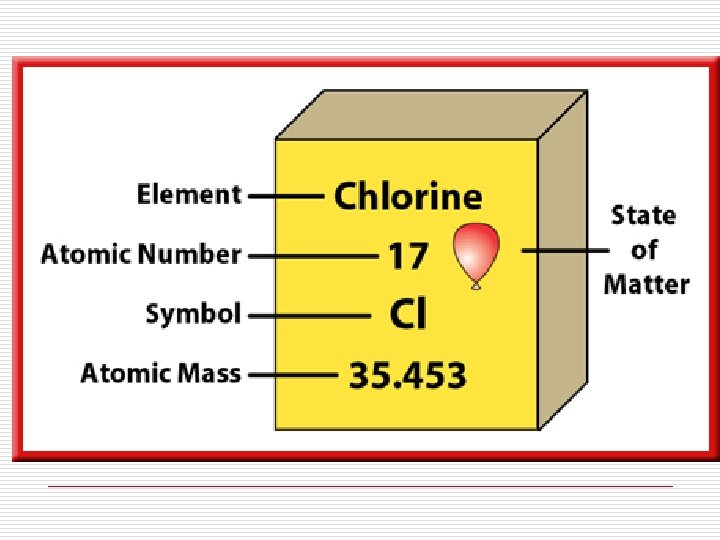Atomic Number Mass Number Atomic Mass and Isotopes

• Slides: 21Atomic Number, Mass Number, Atomic Mass and Isotopes Mrs. Coyle ChemistryAtomic Number (Z): is the number of protons in the nucleus of the atom. Z=#p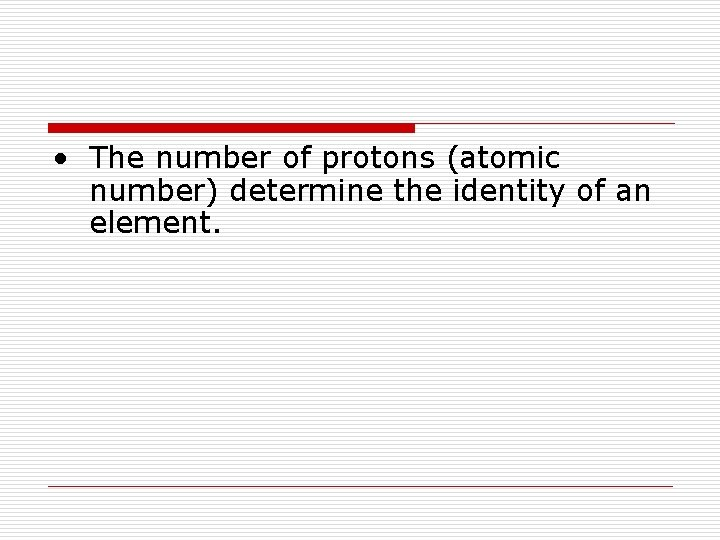• The number of protons (atomic number) determine the identity of an element.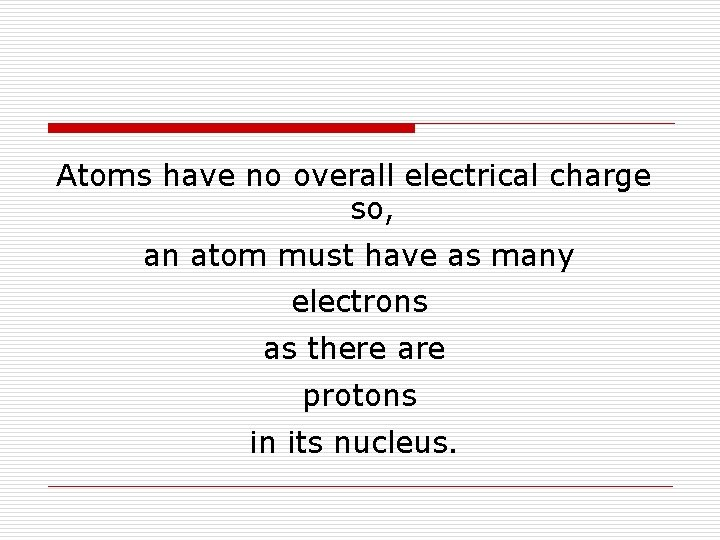Atoms have no overall electrical charge so, an atom must have as many electrons as there are protons in its nucleus.The atomic number of an element also equals the number of electrons in a neutral atom of that element.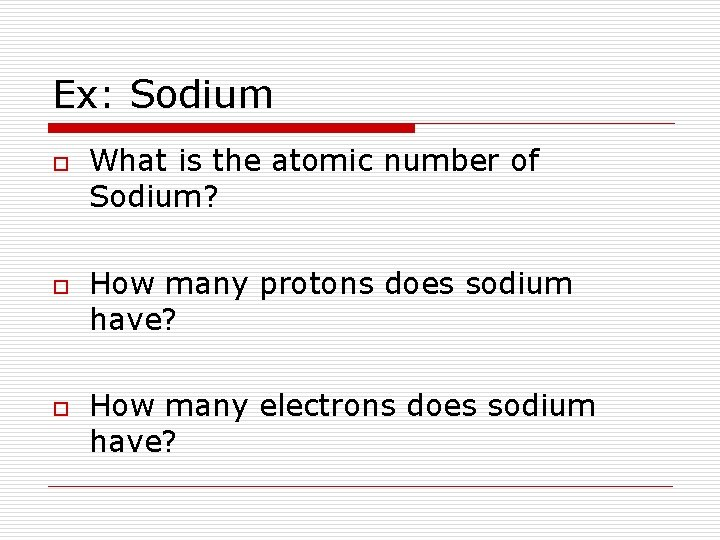Ex: Sodium o o o What is the atomic number of Sodium? How many protons does sodium have? How many electrons does sodium have?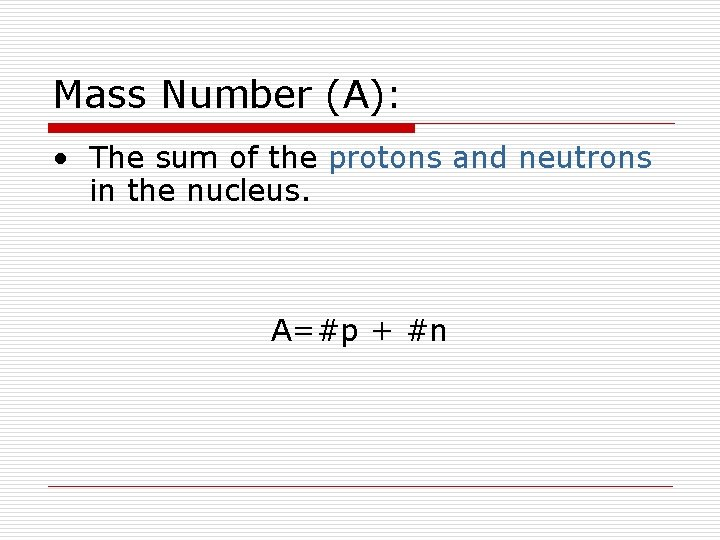Mass Number (A): • The sum of the protons and neutrons in the nucleus. A=#p + #n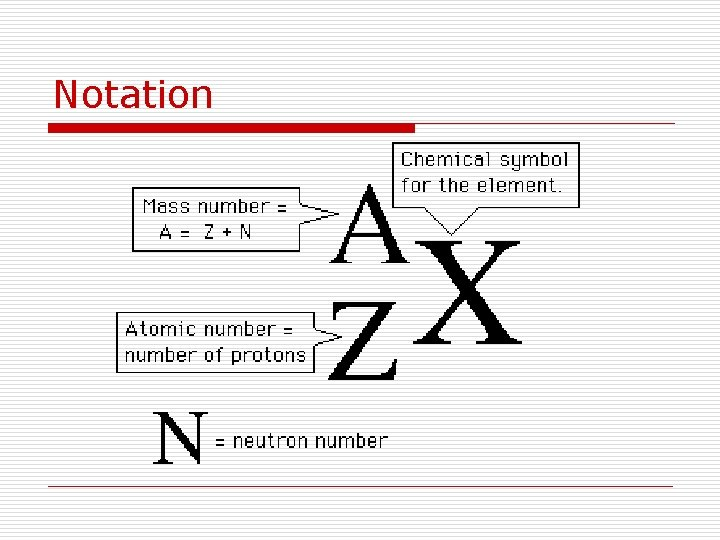Notation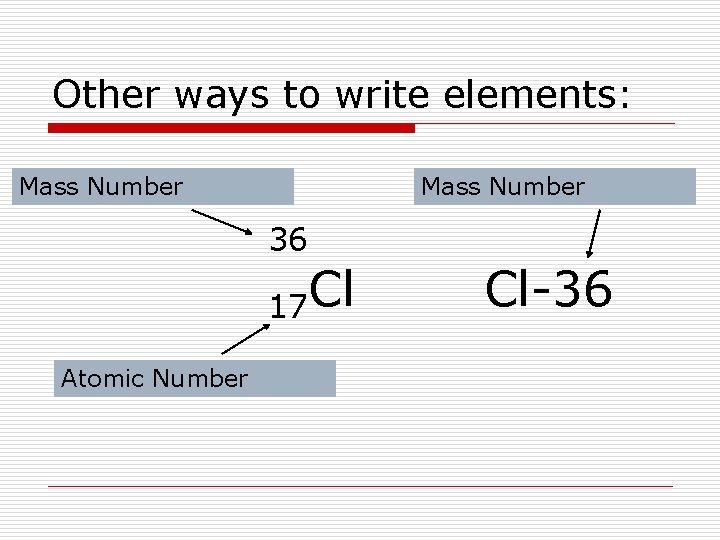Other ways to write elements: Mass Number 36 17 Cl Atomic Number Cl-36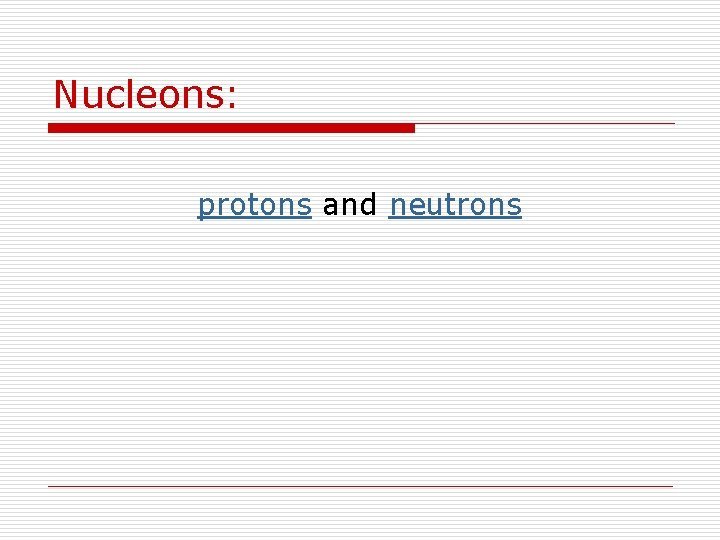Nucleons: protons and neutrons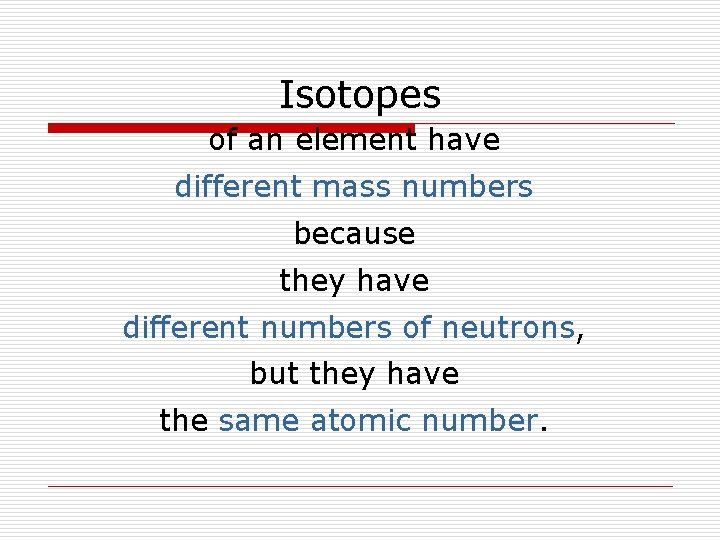Isotopes of an element have different mass numbers because they have different numbers of neutrons, but they have the same atomic number.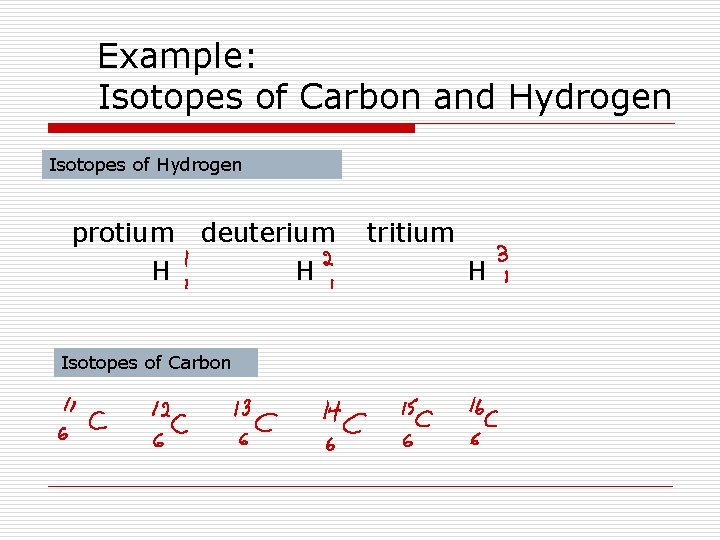Example: Isotopes of Carbon and Hydrogen Isotopes of Hydrogen protium H deuterium H Isotopes of Carbon tritium H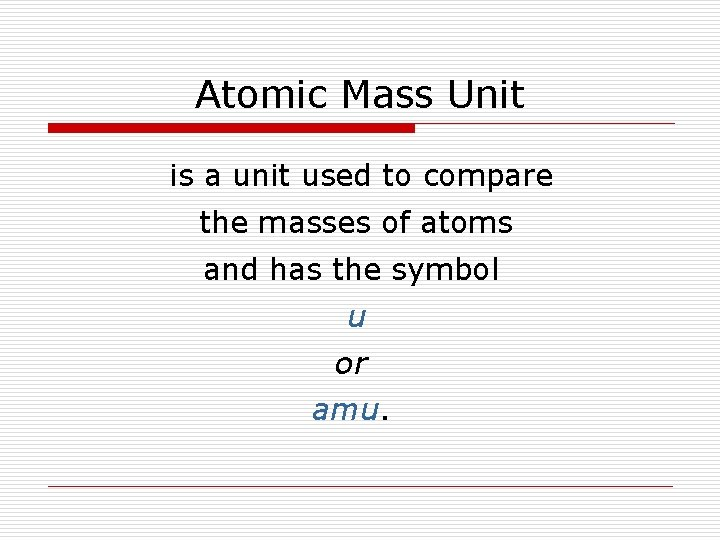Atomic Mass Unit is a unit used to compare the masses of atoms and has the symbol u or amu.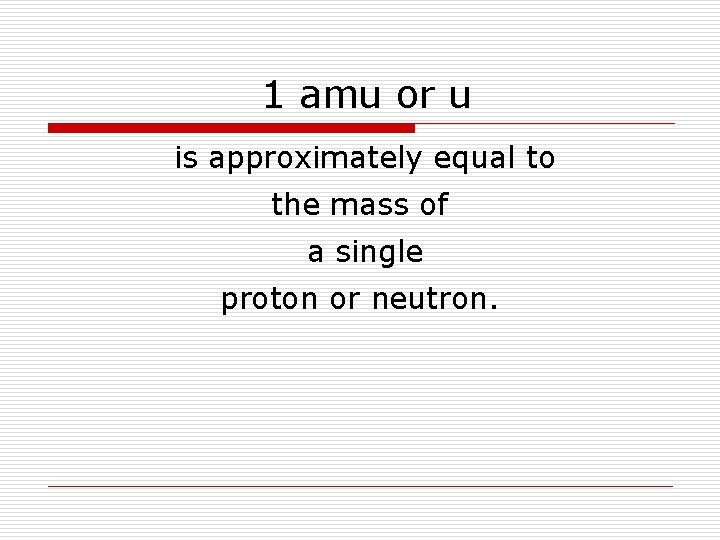1 amu or u is approximately equal to the mass of a single proton or neutron.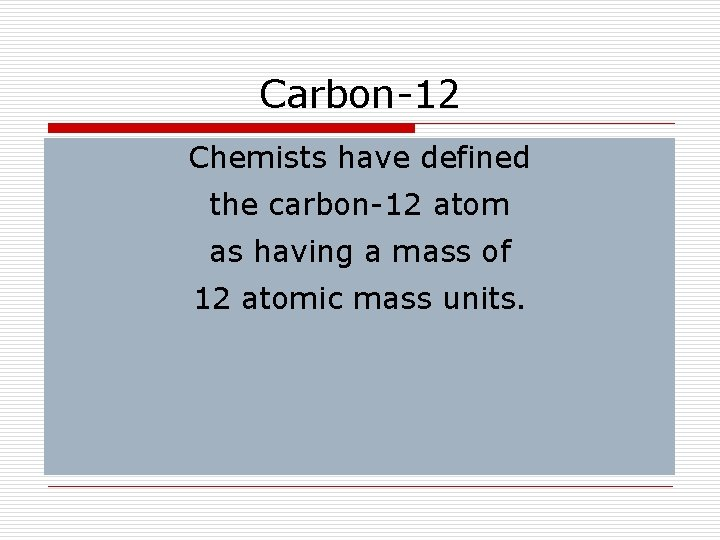Carbon-12 Chemists have defined the carbon-12 atom as having a mass of 12 atomic mass units.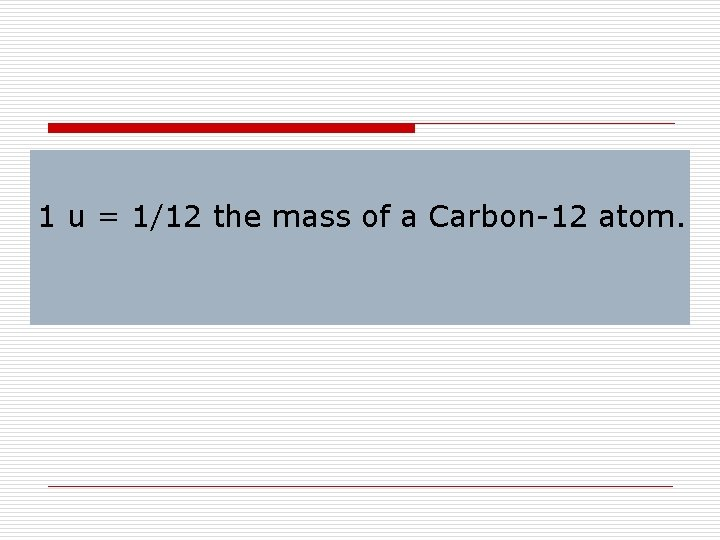1 u = 1/12 the mass of a Carbon-12 atom.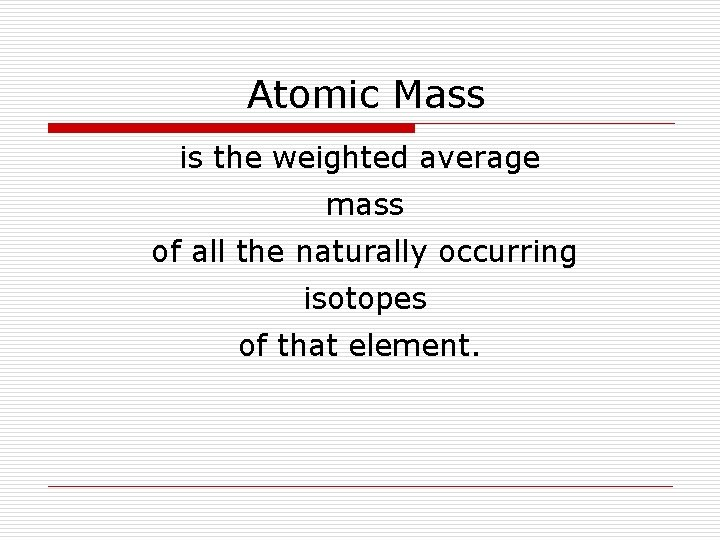Atomic Mass is the weighted average mass of all the naturally occurring isotopes of that element.